# How should we find the average kinetic energy

## The kinetic gas theory¶

Many real gases can be described to a good approximation under standard conditions using the ideal gas model: The forces of attraction between the individual particles are usually negligible, and the volume of the individual particles is also small compared to the total volume of the gas. Based on these assumptions, a gas can be viewed as a large number of individual atoms or molecules that move at different speeds in different directions, with the individual directions of movement and speeds being statistically evenly distributed.

In a simple model, one can assume a single gas particle that is contained in a cubic container in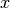-Direction moved towards the left wall of the container. One denotes with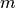the mass of the gas particle and with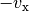its original velocity, then applies to the change in momentum that the particle experiences during an elastic collision with the wall: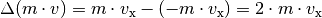Before the particle hits the left wall again, it has to travel a distance of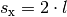put back whenis the length of the cube. The following time thus elapses until the next impact with the left wall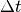: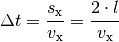The force that the particle exerts on the wall is equal to the change in momentum over time: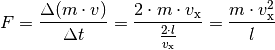The pressure exerted by the individual particle on the left wall is equal to the quotient of the force and the wall area: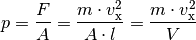In the final calculation step, the relationship was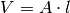used. If one does not start from an individual, but from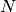Particles that are in-Direction with the speeds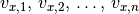move back and forth, the individual contributions add up to the total pressure: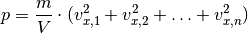Since there are usually a lot of particles in a gas volume, it makes much more sense to use instead ofindividual speed squares with- to be calculated times the mean square of the velocity of the particles. This corresponds to the arithmetic mean of the individual values: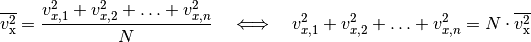Inserting this expression into the above equation, one obtains: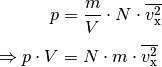When the gas particles move in the container, there is no preferred direction of velocity. If there are on average the same number of gas particles in-,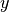- and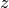-Must move direction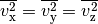be valid.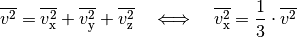Thus, the above equation can be written in the following form: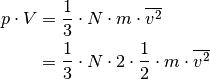In the last calculation step, the term on the right-hand side was with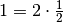multiplied, which leaves the value of the term unchanged, but allows the factor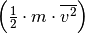as mean kinetic energy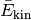to understand the gas particles. If one transforms the equation further, it follows: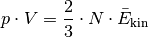The expression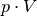on the left side of the equation corresponds to straight according to the general gas equation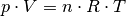. Instead of the general gas constant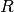can also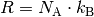be written, where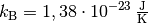is the so-called Boltzmann constant.  For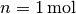of a gas: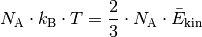Thus, as a result of the kinetic gas theory one finally obtains the following equation:

The kinetic energy of the gas particles increases in direct proportion to the (absolute) temperature of the gas. Equation (1) can be used on the one hand to determine the total kinetic energy of the particles of a gas quantity at a certain temperature, on the other hand it can also be calculated how great the average speed of the individual gas particles is at a certain temperature.

Examples:

• What is the total kinetic energy of all molecules of an ideal gas at a temperature of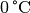and an amount of substance of?

According to the kinetic gas theory, the following applies to the average kinetic energy of a single gas particle: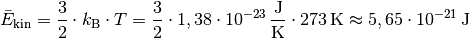Multiply this value by the number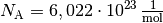of particles per mole, the total energy for one mole of particles is: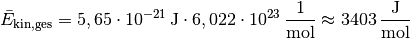Under normal conditions, one mole of an ideal gas takes up a "normal volume" of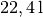a. The kinetic energy contained in it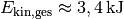of all particles corresponds roughly to the kinetic energy of one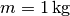heavy object moving at a speed of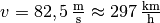emotionally. This considerable amount of energy is, for example, the reason why heat pumps can “tap” part of the kinetic particle energies from a colder environment and transfer it to a warmer environment.

Degrees of freedom of polyatomic gas particles

According to equation (1), the absolute temperature is a measure of the mean kinetic energy of a gas particle. A monatomic gas can move translationally in any of the three directions of space; It is said that such a gas particle has three degrees of translational freedom. In addition, an atomic gas particle can rotate around its center of gravity (similar to a rigid sphere), so it also has three degrees of rotational freedom.

• Monthomic gases can be imagined as "smooth" spheres; they do not exchange any rotational energy in the event of a collision. For monatomic gases, only the three degrees of translational freedom are important.
• Gas particles that consist of two atoms can be imagined as models like “dumbbells”. It has been found experimentally that in such molecules under normal conditions, in addition to the three degrees of translational freedom, only two Degrees of freedom of rotation appear: At normal temperatures, the molecule can rotate around both transverse axes, but not around the longitudinal axis; the third degree of rotational freedom is called "frozen".  All in all, diatomic gas particles show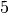Degrees of freedom.
• Triatomic gases, the atoms of which do not lie on a common straight line, and polyatomic gases have three (or even more) degrees of rotational freedom.

For the kinetic gas theory, the degrees of freedom of the gas particles are important in that it has been determined that the mean kinetic energy of an ideal gas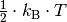per mole and degree of freedom is:

Here is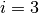for monatomic gases,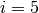for diatomic gases and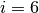for three and polyatomic gases.

Example:

• What is the root of the mean square of the speed?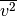of air molecules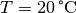?

According to the kinetic gas theory, the average kinetic energy of a single gas particle is the mass: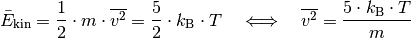As shown in the Particle Number and Molar Mass section, the mass of one mole of nitrogen particles is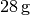, the mass of one mole of oxygen particles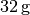. If you divide these values ​​by the numberof particles per mole, the mass of a nitrogen or oxygen particle is: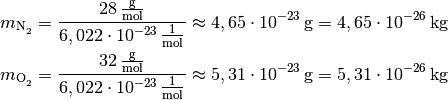If you insert these values ​​into the above equation, you get for the velocities of the nitrogen and oxygen molecules: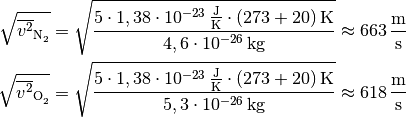The nitrogen particles are round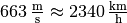thus faster than the oxygen particles with around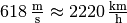.

The lower the molar mass of a gas, the higher the average speed of the particles it contains at a certain temperature.

Maxwell's velocity distribution

The speed values ​​of the individual gas particles are statistically distributed, with the distribution of all speeds occurring using a distribution functioncan be specified. Such a function indicates the frequency with which a number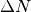AllesGas particles have a speed between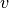other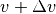owns: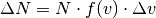In the case of gas particles, the frequency distribution does not correspond to a normal distribution, but to a so-called Maxwellian distribution. The following diagram shows this distribution function for three different temperatures.

The speed that corresponds to the maximum of each curve is considered the most likely speed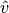designated; it does not agree with the medium speed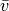of all speed values.

... to be continued ...

Remarks: